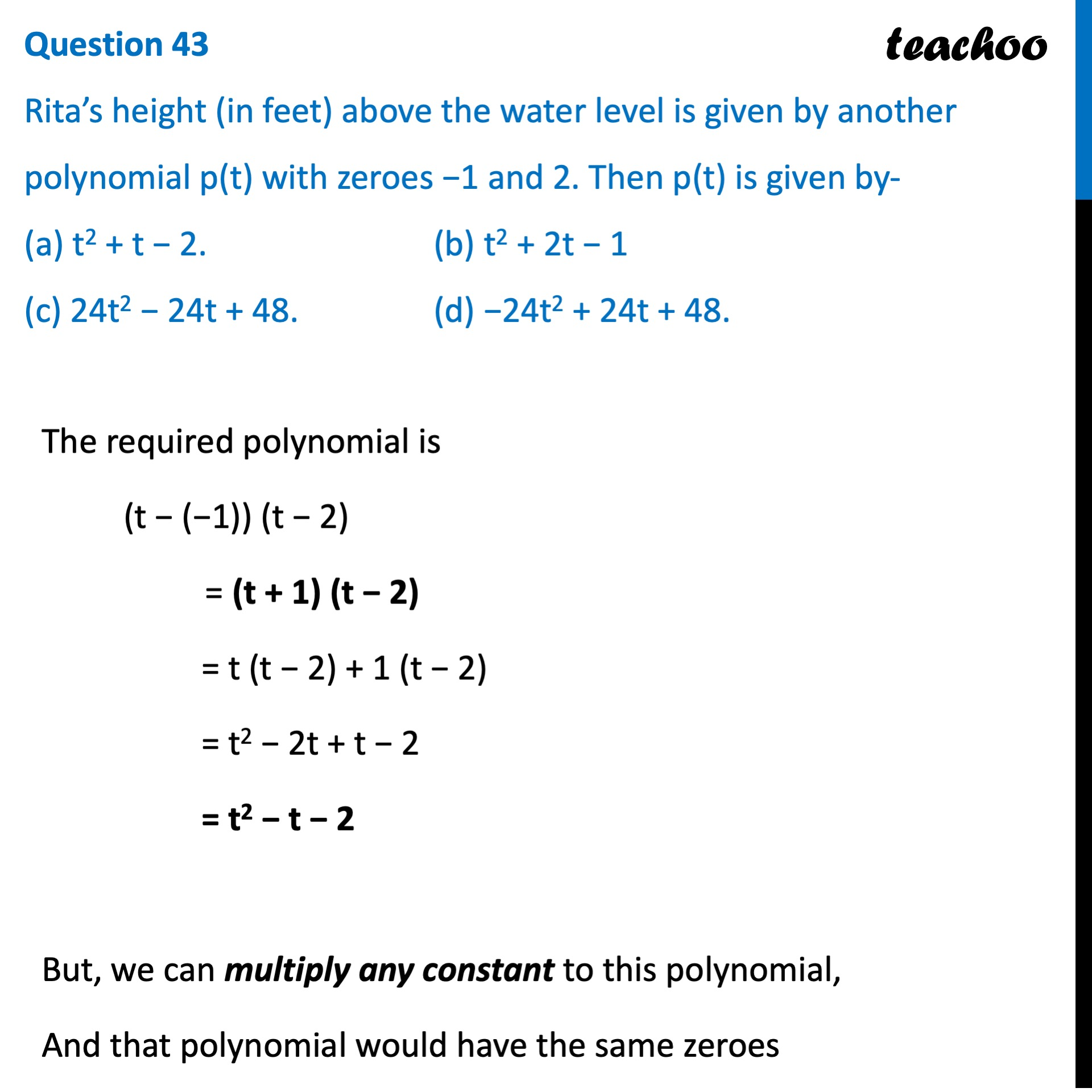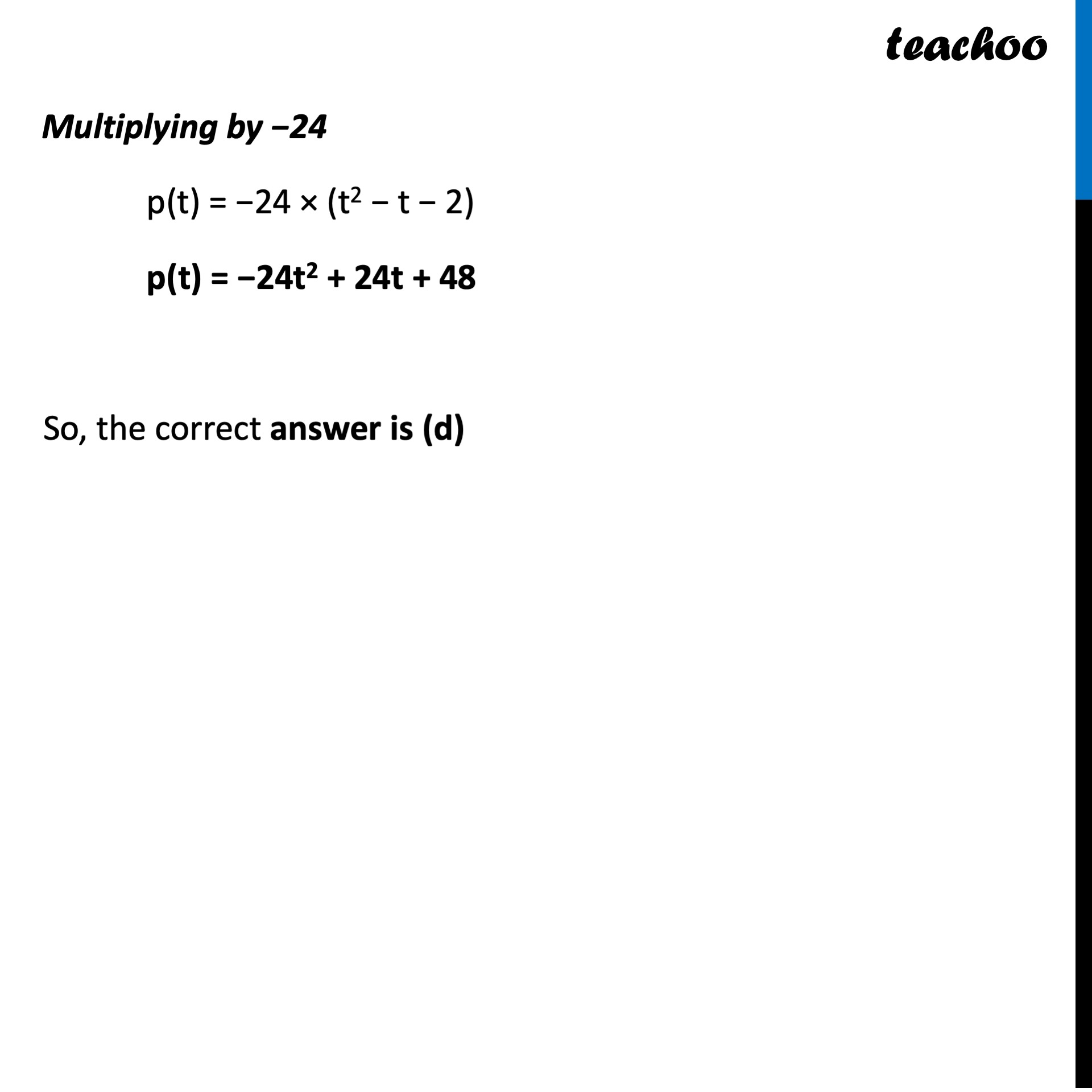CBSE Class 10 Sample Paper for 2022 Boards - Maths Standard [MCQ]

Class 10
Solutions of Sample Papers for Class 10 Boards

## (c) 24t 2 − 24t + 48.   (d) −24t 2 + 24t + 48.Learn in your speed, with individual attention - Teachoo Maths 1-on-1 Class

### Transcript

Question 43 Rita’s height (in feet) above the water level is given by another polynomial p(t) with zeroes −1 and 2. Then p(t) is given by- (a) t2 + t − 2. (b) t2 + 2t − 1 (c) 24t2 − 24t + 48. (d) −24t2 + 24t + 48. The required polynomial is (t − (−1)) (t − 2) = (t + 1) (t − 2) = t (t − 2) + 1 (t − 2) = t2 − 2t + t − 2 = t2 − t − 2 But, we can multiply any constant to this polynomial, And that polynomial would have the same zeroes Multiplying by −24 p(t) = −24 × (t2 − t − 2) p(t) = −24t2 + 24t + 48 So, the correct answer is (d)